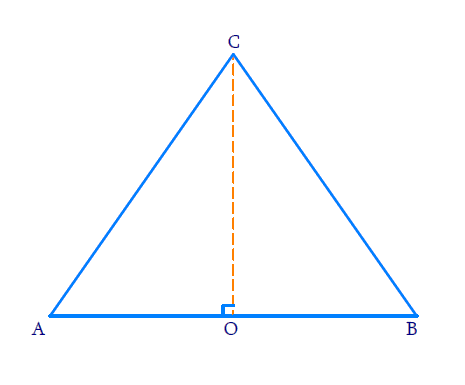# Ex.8.1 Q6 Introduction to Trigonometry Solution - NCERT Maths Class 10

Go back to  'Ex.8.1'

## Question

If $$\,\,\angle {A}$$ and $$\,\,\angle {B}$$ are acute angles such that $$\,\,\text{cos}\,{A}=\text{cos}\,{B},$$ then show that $$\angle {A}=\angle \,{B}\,{.}$$

Video Solution
Introduction To Trigonometry
Ex 8.1 | Question 6

## Text Solution

#### What is the known?

$$\angle {A}$$ and $$\,\,\angle {B}$$ are acute angles and \begin{align}\text{cos}\, A = \text{cos}\, B\end{align}

#### What is the unknown?

To show that $$\angle A= \angle B$$

#### Reasoning:

Using $$\text{cos} \,A$$ and $$\text{cos}\, B,$$ we can find the ratio of the length of two sides of the right-angled triangle with respective angles. Then compare both the ratios.

#### Steps:

In the right-angled triangle $$ABC, \,$$$$\,\angle A$$  and  $$\angle B$$ are acute angles and $$\angle C$$ is right angle.

\begin{align} & \text{cos}\,{A}\,{=}\,\frac{\text{side adjacent to}\ \angle {A}}{\text{hypotenuse}}{=}\frac{{AC}}{{AB}} \\ & \text{cos}\,{B}\,{=}\,\frac{\text{side adjacent to}\,\angle {B}}{\text{hypotenuse}}{=}\,\frac{{BC}}{{AB}} \end{align}

Given that \begin{align} \;\text{cos} \,A&=\,\text{cos} B \end{align}

\begin{align} \text{Therefore, }\frac{{AC}}{{AB}}\,&=\,\frac{{BC}}{{AB}} \\ {AC}\,&=\,{BC}\end{align}

Hence, $$\angle A=\angle B\,,$$ (angles opposite to equal sides of triangle are equal)

Alternatively,

Reasoning:

Using $$\text{ cos} \,A$$ and $$\text{cos}\, B,$$ we can find the ratio of the length of two sides of the right-angled triangle with respective angles. Then by using Pythagoras theorem, relation between the sides

Let us consider a triangle $$ABC$$ in which \begin{align}{CO} \perp {AB}\end{align}It is given that

\begin{align} \text{cos}\,{A}\,&=\, \text{cos}\,{B} \\ \frac{{AO}}{{AC}}&=\frac{{BO}}{{BC}} \\ \frac{{AO}}{{BO}}\,&=\,\frac{{AC}}{{BC}}\\ \\ \text{ Let }\; \frac{ {A O}}{{B O}}&=\frac{{A C}}{{B C}}={k} \\ { A O}&={k B O}\;\dots\rm{(i)} \\ {A C}&={k B C}\;\dots \rm{(ii)} \end{align}

By applying Pythagoras theorem in  $$\Delta {CAO}$$ and $$\Delta {CBO},$$ we get.

\begin{align} {A}{{{C}}^{{2}}} & ={A}{{{O}}^{{2}}}{+C}{{{O}}^{{2}}}[\text{from} \;\Delta\;{ CAO}] \\ {C}{{{O}}^{{2}}}&={A}{{{C}}^{{2}}}-{A}{{{O}}^{{2}}}\cdots \left(\rm {iii} \right) \\ {B}{{{C}}^{{2}}}&={B}{{{D}}^{{2}}}{+C}{{{O}}^{{2}}}[\text {from}\;\Delta\,{ CBO}] \\ {C}{{{O}}^{{2}}}&={B}{{{C}}^{{2}}}\,-{B}{{{O}}^{{2}}}\cdots \left( \rm{iv} \right) \\ \end{align}

From equation (iii) and equation (iv), we get

\begin{align} {A}{{{C}}^{{2}}}-{A}{{{O}}^{{2}}}&=\,{B}{{{C}}^{{2}}}-{B}{{{O}}^{{2}}} \\ \ {{{(kBC)}}^{{2}}}-{{{(kBO)}}^{{2}}}&=\,{B}{{{C}}^{{2}}}-{B}{{{O}}^{{2}}} \\ {{k}^{{2}}}{B}{{{C}}^{{2}}}-{{k}^{{2}}}{B}{{{O}}^{{2}}}\,&=\,{B}{{{C}}^{{2}}}-{B}{{{O}}^{{2}}} \\ {{k}^{{2}}}{(B}{{{C}}^{{2}}}-{B}{{{O}}^{{2}}}{)}\,&=\,{B}{{{C}}^{{2}}}-{B}{{{O}}^{{2}}} \\ {{k}^{{2}}}\,&=\,\frac{{B}{{{C}}^{{2}}}-{B}{{{O}}^{{2}}}}{{B}{{{C}}^{{2}}}-{B}{{{O}}^{{2}}}} \\ &=1 \\k\,&=1 \end{align}

Putting this value in equation (ii) we obtain

${AC}\,{=}\,{BC}$

$${\angle A=\angle B}$$ (angles opposite to equal sides of triangle are equal.)

Learn from the best math teachers and top your exams

• Live one on one classroom and doubt clearing
• Practice worksheets in and after class for conceptual clarity
• Personalized curriculum to keep up with school